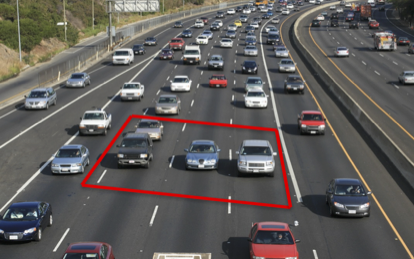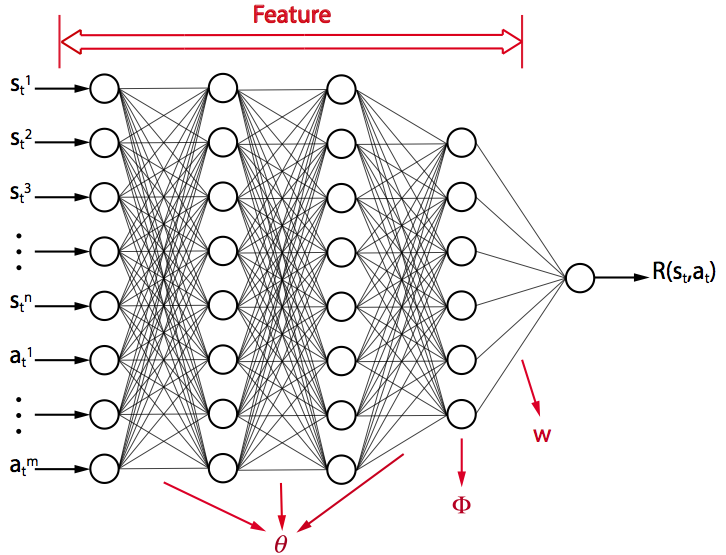## Advanced Planning for Autonomous Vehicles Using Reinforcement Learning and Deep Inverse Reinforcement Learning

Autonomous vehicles promise to improve traffic safety while, at the same time, increase fuel efficiency and reduce congestion. This project concentrates on the path planning problem of autonomous vehicles in traffic, as shown in Figure 1. Each vehicle has a couple of actions to take, such as maintaining its current speed, switching to the left/right lane, speeding up and braking. We model the interaction between the autonomous vehicle and the environment as a stochastic Markov decision process (MDP).Figure 1: The traffic on multi-lane road.

The state of the MDP is defined using the positions of the autonomous vehicle, and the number and positions of the environmental vehicles around the automnous vehicle. The road geometry is taken into consideration in the MDP model in order to incorporate more diverse driving styles.Figure 2: The cells and the definition of the state: (1) 9-cell internal-lane state, (2) 6-cell left-boundary state and (3) 6-cell right-boundary state

The core problem of an MDP is to find a policy $\pi$ for the agent, where the policy $\pi:S\rightarrow A$ specifies the action to take at the current state $s_t$. The goal is to find the optimal policy $\pi^*$ that maximizes the cumulative discounted reward over an infinite horizon: \begin{align} \label{eqn:OCP} \ \pi^* = \arg \max\limits_{\pi} ~ \mathbb{E}\Big[\sum_{t=0}^{\infty} \gamma^t R(s_t,\pi(s_t))\Big], \end{align} The desired, expert-like driving behavior of the autonomous vehicle can be obtained using two approaches.

First, we design the reward function of the corresponding MDP and determine the optimal driving strategy for the autonomous vehicle using reinforcement learning techniques.

Second, we collect a number of demonstrations from an expert driver and learn the optimal driving strategy based on data using inverse reinforcement learning. The unknown reward function of the expert driver is approximated using a deep neural-network (DNN).Figure 3: Deep neural-network feature function and reward.

Movies for demonstrating the typical driving styles such as overtaking and tailgating are available here and here.